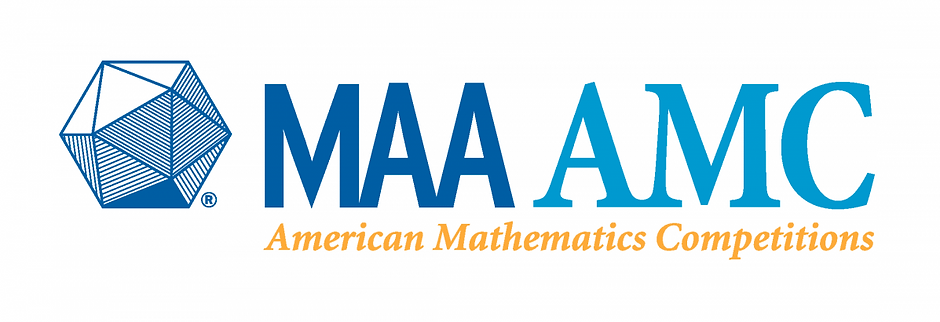top of page# AMC 8

The AMC 8 is a 25-question, 40-minute, multiple choice examination in middle school mathematics designed to promote the development of problem-solving skills. The AMC 8 provides an opportunity for middle school students to develop positive attitudes towards analytical thinking and mathematics that can assist in future careers. Students apply classroom skills to unique problem-solving challenges in a low-stress and friendly environment.

The material covered on the AMC 8 includes topics from a typical middle school mathematics curriculum. Possible topics include but are not limited to: counting and probability, estimation, proportional reasoning, elementary geometry including the Pythagorean Theorem, spatial visualization, everyday applications, and reading and interpreting graphs and tables. In addition some of the later questions may involve linear or quadratic functions and equations, coordinate geometry, and other topics traditionally covered in a beginning algebra course.

AMC8 Intensive Summer Prep Course.

Curriculum Outline

Counting and Number System

• Number Patterns

• Sequence and Series

• Operations with Fractions

• Operations with Decimals

• Even and Odd

• Counting Techniques

• Divisibility

• Factors

• Remainder

• Prime Numbers

• Least Common Multiple (LCM) and Greatest Common Factor (GCF)

Proportional Reasoning

• Ratio, Rate and Proportion

• Word Problems related to Percentage

Geometry

• Perimeter and Area

• Transformation

• Geometric Visualization

• Pythagorean Theorem and Triangles

• Angles and Triangles

• Rectangles and Squares

• Similar Triangles

• Trapezoids

• Circles

• Volumes

Functions

• Linear Equations

• Special Equations

Statistics and Probabilities

• Basic Probability Formula

• General Addition Rule and General Multiplication Rule

• General Geometric Probability Formula

bottom of page